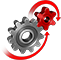# WolframSystemModeler

Binary adders are digital circuits often used in computers for basic arithmetic operations. Using the digital library in the standard Modelica library, we have constructed an 8-bit adder that takes two 8-bit integers and calculates their sum.

To run this example, you'll need

The 8-bit adder adds the numbers digit by digit, as can be seen in the schematic diagram below. In this example, the integers 170 and 51 represent input a and b, respectively, and the resulting output is the sum 221. The first adder does not have any carry‐in, and so it is represented by a half adder (HA) instead of a full adder (FA).This schematic diagram explains the principle of using half and full adders to calculate the sum of two 8-bit integers.
Going down one level in the calculator model, we can see the eight full adders where the output, c, of one adder constitutes the input, cin, of another. The rightmost adder is in practice a half adder, since the SET component gives a carry‐in of 0. The other adder inputs, denoted a and b, are binary digits that are converted from the original integers. The last step at the bottom of the diagram shows the conversion of the binary result to the final integer sum.

### Simulation ResultThe input to the calculator is varied using IntegerTable from the Modelica Standard Library. For each set of integers, the sum is calculated and simulated.

Build a model of a calculator

The half and full adders in the Modelica Digital library can be used to construct a calculator for addition of integer numbers.

## Wolfram System Modeler

Questions? Comments? Contact a Wolfram expert »